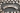# Intro to Variable Expressions

POSTED ON APRIL 15, 2020## Lesson Intro: Expressions with Variables

In this lesson, Juni Mathematics instructor Kadyn will be teaching you about variables and variable expressions - foundational concepts in pre-algebra.

Variables are important to know for higher levels of math, and are even used similarly in other subjects like computer science! Keep reading to learn more about what variables are and how to use them in expressions.

Finally, put what you've learned to practice below with instructor Kadyn's warmup questions, drills, and word problems worksheets for variable expressions. We've provided an answer key for every worksheet, along with some tips for solving various types of problems.

## What are Variables?

### Key Terms:

• Variable
• A variable is a letter that represents an unknown number. Variables are commonly represented by the letter x, but can also be represented by other letters like y, z, and so on.

• Coefficient
• When there is a number in front of a variable, that number is called the coefficient of that variable. A coefficient represents how many times we have a specific variable.

For example, 3x means we have three x's, which can also be written as x + x + x. That expression can then be simplified to 3 ⋅ x = 3x.

If the variable doesn't have a number attached to it, its coefficient is 1. That means: x = 1x = 1 ⋅ x.

• Like terms
• When we have like terms, meaning terms with the same variable, we can combine them and add the coefficients together. For example, 3x + x = 3x + 1x = (3 + 1) ⋅ x = 4x.

However, terms with different variables cannot be combined. For instance, we cannot add x and y because they are two different variables representing two different unknown numbers.

## Solving Equations with Unknown Variables

Now that we're more familiar with what variables are and how we can use them in expressions, let's talk about different approaches we can take to solve for unknown variables.

When we face math problems talking about variables, we are typically asked to find what an unknown variable is by writing an equation. When solving equations with unknown variables, there are two ways to approach the problem:

1. Guessing and Checking
2. Isolating the Variable

### Guessing and Checking

Guessing and checking challenges us to make an educated guess for what number can replace the variable to satisfy the equation. For example, if we have 3x = 12, we can start substituting x with numbers from 1, 2, and so on until we eventually get our answer x = 4.

A helpful way to use this approach is to get a small range of numbers that our answer can exist in between, and substitute each number in that range until we find our answer that makes the equation true.

### Isolating the Variable

Isolating the variable is a very useful way to solve equations with unknown variables. This requires us to keep only the variable on one side of the equal sign and move everything else to the other side. Once we have the variable isolated, we have to modify the coefficient so that we eventually have the form x = _. We will then have figured out what x is.

This method usually requires practice and time to understand. But once mastered, it is the best way to solve equations with variables.

## Practice Worksheets: Test Your Knowledge

If you feel comfortable with the key terms and question approaches above, try challenging yourself with Kadyn's Intro to Variable Expressions Worksheets!

Start with some warmups to solidify your understanding, review, or jog your brain to get into math learning for the day. Then, master your skills with some more challenging drill questions that target specific concepts taught in this lesson. Finally, try applying these concepts more broadly by tackling word problems involving variables. Solutions and walkthroughs are provided at the bottom of each worksheet.

## Need help or want to keep learning?

We hope you enjoyed Instructor Kadyn's Lesson and Worksheets on Variable Expressions! This lesson falls under our Pre-Algebra A course curriculum.

To keep practicing or learning, please check out all of our math and coding tutorials on our Math Practice page.

Interested in learning more about Juni's [online math courses](https://www.junilearning.com/paths/math)? Speak with a Juni Advisor today by calling __(650) 263-4306__ or emailing __advisors@learnwithjuni.com__.